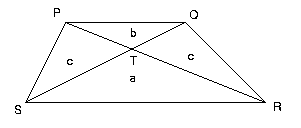#### You may also like### Golden Thoughts

Rectangle PQRS has X and Y on the edges. Triangles PQY, YRX and XSP have equal areas. Prove X and Y divide the sides of PQRS in the golden ratio.### From All Corners

Straight lines are drawn from each corner of a square to the mid points of the opposite sides. Express the area of the octagon that is formed at the centre as a fraction of the area of the square.### Star Gazing

Find the ratio of the outer shaded area to the inner area for a six pointed star and an eight pointed star.

# Same Height

##### Age 14 to 16 Challenge Level:

A trapezium is divided into four triangles by its diagonals. Suppose the two triangles containing the parallel sides have areas a and b , what is the area of the trapezium?

The following solution was done by Ling Xiang Ning, Allan from Tao Nan School, SingaporeFirst note that triangles SPR and SQR are equal in area (same base and height) so triangles SPT and RQT are equal in area; suppose this area is c .

Now triangles SPT and TPQ have the same height (with their common base on SQ) and the ratio of their areas is:
$$\frac{c}{b} = \frac{\text{Area}(SPT)}{\text{Area}(TPQ)} = \frac{ST}{TQ}$$
$$\frac{a}{c} = \frac{\text{Area}(SRT)}{\text{Area}(TRQ)} = \frac{ST}{TQ}$$
hence $c/b = a/c$.

Then $c^2 = ab$ and $c = \sqrt{ab}$

Therefore, the total area of the trapezium is $a + b + 2\sqrt{ab}$.